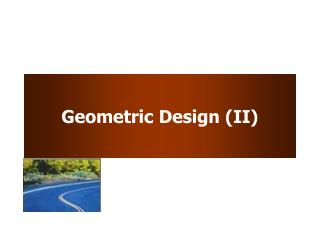Download PresentationGeometric Design (II)

# Geometric Design (II) - PowerPoint PPT Presentation

Geometric Design (II). Learning Objectives. To calculate minimum radius of horizontal curve To understand design concepts for transition curves and compute min length To understand the role of SSD in horizontal and vertical design To define and apply grade considerationsI am the owner, or an agent authorized to act on behalf of the owner, of the copyrighted work described.
Download Presentation## Geometric Design (II)

Download Policy: Content on the Website is provided to you AS IS for your information and personal use and may not be sold / licensed / shared on other websites without getting consent from its author.While downloading, if for some reason you are not able to download a presentation, the publisher may have deleted the file from their server.

- - - - - - - - - - - - - - - - - - - - - - - - - - E N D - - - - - - - - - - - - - - - - - - - - - - - - - -
Presentation Transcript
1. Geometric Design (II)

2. Learning Objectives • To calculate minimum radius of horizontal curve • To understand design concepts for transition curves and compute min length • To understand the role of SSD in horizontal and vertical design • To define and apply grade considerations • To develop vertical curves (Chapter 6.1 ~ 6.4)

3. Horizontal Curve • Minimum Curve Radius • Curve requiring the most centripetal force for the given speed • Given emax, umax, Vdesign R

4. Point of Tangency Point of Curvature Horizontal Curve Properties Based on circular curve • R: radius of curve • D: degree of curve • : central angle • T: length of tangent • L: length of curve • LC: long chord • M: middle ordinate dist • E: external dist

5. Horizontal Design Iterations • Design baseline • Curve radius above the minimum • Superelevation and side-friction factor not exceeding the maximum values • Design is revised to consider: cost, environmental impacts, sight distances, aesthetic consequences, etc.

6. R Horizontal Curve Sight Distance • Sight line is a chord of the circular curve • Sight Distance is curve length measured along centerline of inside lane

7. Horizontal Curve Sight Distance Figure 6-10

8. Transition Curves • Gradually changing the curvature from tangents to circular curves Without Transition Curves With Transition Curves

9. Transition Curves • Gradually changing the curvature from tangents to circular curves • Use a spiral curve L: min length of spiral (ft) V: speed (mph) R: curve radius (ft) C: rate of increase of centrifugal accel (ft/sec3), 1~3

10. Transitional Curves • Gradually changing the cross-section of the roadway from normal to superelevated (Figure 6-9) Keep water drainage in mind while considering all of the available cross-section options

11. Vertical Alignment Reduced Speed Increased Speed

12. Vertical Alignment • Grade • measure of inclination or slope, rise over the run • Cars: negotiate 4-5% grades without significant speed reduction • Trucks: significant speed changes • 5% increase on short descending grades • 7% decrease on short ascending grades

13. Grade Considerations • Maximum grade – depends on terrain type, road functional class, and design speed Rural Arterials

14. Grade Considerations • Critical length of grade • Maximum length which a loaded truck can travel without unreasonable speed reduction • Based on accident involvement rates with 10mph speed reduction as threshold

15. Grade Considerations General Design Speed Reduction

16. Vertical Curves • To provide transition between two grades • Consider • Drainage (rainfall) • Driver safety (SSD) • Driver comfort • Use parabolic curves • Crest vs Sag curves

17. Vertical Curves

18. Vertical Curves • Given • G1, G2: initial & final grades in percent • L: curve length (horizontal distance) • Develop the actual shape of the vertical curve point of vertical intersection PVI point of verticaltangency point of vertical curvature G1% G2%

19. Vertical Curves • Define curve so that PVI is at a horizontal distance of L/2 from PVC and PVT • Provides constant rate of change of grade: A G1% G2%

20. Example • G1 = 2% • G2 = -4% • Design speed = 70 mph • Is this a crest or sag curve? • What is A?

21. Vertical Curves • Major control for safe operation is sight distance • MSSD should be provided in all cases (use larger sight distance where economically and physically feasible) • For sag curves, also concerned with driver comfort (large accelerations due to both gravitational and centrifugal forces)

22. Crest Vertical Curves • Critical length of curve, L, is where sight line is tangent to the crest • Assume driver eye height (H1) = 3.5 ft and object height (H2) = 2.0 ft and S=MSSD

23. Sag VC - Design Criteria • Headlight sight distance • Rider comfort • Drainage control • Appearance